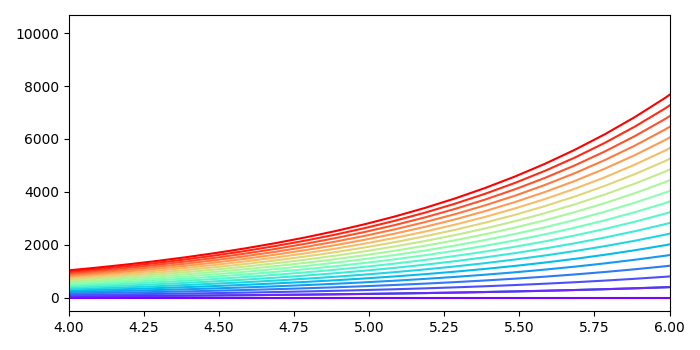# Matplotlib Plot Lines with Colors through Colormap

To plot lines with colors through colormap, we can take the following steps−

• Create x and y data points using numpy
• Plot x and y data points using plot() method.
• Count n finds, number of color lines has to be plotted.
• Iterate in a range (n) and plot the lines.
• Limit the x ticks range.
• Use show() method to display the figure.

## Example

import numpy as np
import matplotlib.pylab as plt
plt.rcParams["figure.figsize"] = [7.00, 3.50]
plt.rcParams["figure.autolayout"] = True
x = np.linspace(0, 2 * np.pi, 64)
y = np.exp(x)
plt.plot(x, y)
n = 20
colors = plt.cm.rainbow(np.linspace(0, 1, n))
for i in range(n):
plt.plot(x, i * y, color=colors[i])
plt.xlim(4, 6)
plt.show()

## Output